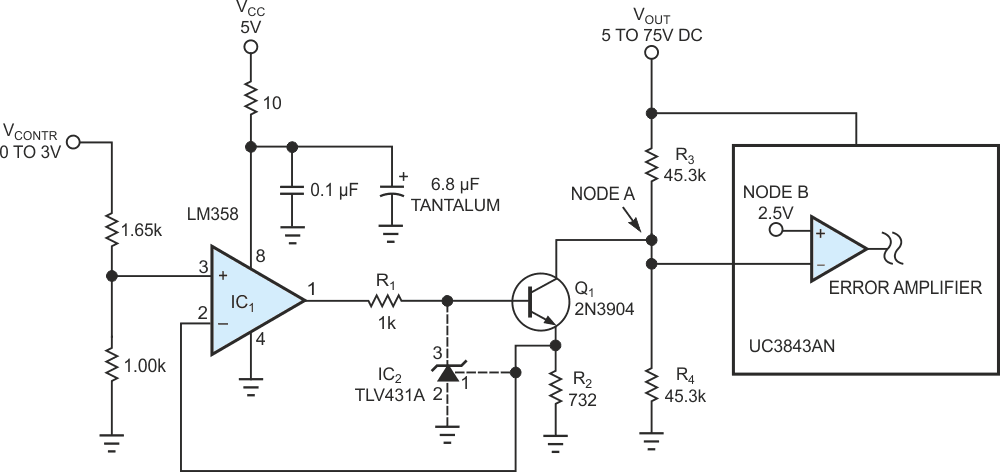# Shunt regulator provides overvoltage protection

## Texas Instruments UC3843AN TLV431A LM358

The circuit in Figure 1 uses a typical technique for varying the output voltage of a power supply via a programmable control voltage. Although the topology and schematic details of the power supply are not critical, the protection technique is novel.Figure 1. Adding one shunt regulator, IC2, to an otherwise typical programmable power supply provides precise overvoltage protection.

The control IC is a UC3843AN PWM controller. This IC applies 2.5 V to the noninverting input of an internal error amplifier but does not bring this input out to a pin (node B). The inverting input of the error amplifier is available at an external pin (node A). To regulate VOUT, the control IC must maintain the voltage at Node A equal to the 2.5 V at Node B. The component values in the figure allow the dc output voltage of the power supply to vary between a minimum of 5 V and a maximum of 75 V, as a function of VCONTR, which can vary from 0 to 3 V (corresponding to a VOUT of 5 V and 75 V, respectively).

In the absence of Q1, VOUT and the voltage division of R3 and R4 determine the voltage at Node A. The circuit compares this voltage with the 2.5 V at Node B. The power supply’s output-voltage control loop keeps the voltage at Node A equal to Node B by appropriately controlling VOUT.

The circuit provides programmability of VOUT by sinking current from Node A. VOUT must source any current that flows from this node. Also, the current must flow through R3, which causes the voltage drop across R3 to increase. VOUT is then always equal to the voltage drop across R3 plus a current set by VCONTR. IC1’s op amp forces the voltage across R2 to equal the voltage at Pin 3 of IC1.

The addition of just one component, IC2, adds precise overvoltage protection to the variable-output power supply. IC2 is a low-voltage shunt regulator that incorporates an internal 1.24 V precision reference. This low reference voltage allows you to use this protection circuit with conventional power-supply control ICs for which 2.5 V is a common internal reference voltage. Under normal operating conditions (for output voltages between 5 and 75 V), IC2 does nothing. The voltage at IC2’s reference (Pin 1) is less than its internal 1.24 V reference, so its cathode (Pin 3) draws no current. In this case, IC1 solely controls the voltage at the base of Q1. For example, if VCONTR is 3 V, then the voltage across R2 is 1.13 V and VOUT equals 75 V. Note that, to simplify this example, the beta of Q1 is assumed to be infinite.

However, in the event of any kind of fault that might cause the voltage across R2 to rise above 1.24 V (which corresponds to a maximum VOUT of 81.7 V), the shunt regulator begins to function. As the voltage at Pin 1 of IC2 begins to exceed the internal 1.24 reference voltage, the cathode of IC2 begins to conduct. The cathode of IC2 then pulls down on the base of Q1 to maintain 1.24 V at Pin 1 of IC2. As this happens, IC2 through Q1 controls the voltage across R2. This control causes the output of IC1 to saturate at a positive voltage of approximately 3.7 V because IC1 can no longer keep the voltage across R2 equal to the voltage at its input (Pin 3). The benefit to circuit operation is that IC2 now operates with a constant-cathode current determined by the voltage across R1, which equals (3.7 V – 1.8 V)/ 1 kΩ = 1.9 mA. This level of cathode current ensures that IC2 regulates properly.

This protection circuit is immune to any potential failure mode of IC1’s op amp or the programming voltage source, VCONTR. IC1 operates only from 5 V. If its output (Pin 1) shorts to ground, the minimum VOUT results. If its output shorts to VCC, IC2 sinks (5 V – 1.8 V)/ 1 kΩ = 3.2 mA, and VOUT clamps at the maximum of 81.7 V.

EDN